# Calculus 1 : How to find continuous on the interval by graphing functions

## Example Questions

### Example Question #1 : How To Find Continuous On The Interval By Graphing Functions

On which of the following intervals is the function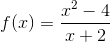continuous?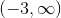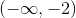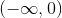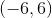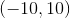Explanation:

The function has a removable discontinuity at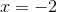.

Since this function is undefined atis it not continuous across any interval containing.

Notice that the correct answer is an open interval that goes up to, but does not include.

### Example Question #1 : How To Find Continuous On The Interval By Graphing Functions

Describe the function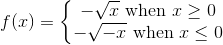on the interval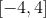.

Non-continuous; Non-differentiable

Non-continuous; Differentiable

Continuous; Non-differentiable

Continuous; Differentiable

Continuous; Non-differentiable

Explanation:

This function (shown below) is defined for every value along the interval with the given conditions (in fact, it is defined for all real numbers), and is therefore continuous.  However, there is a cusp point at (0, 0), and  the function is therefore non-differentiable at that point.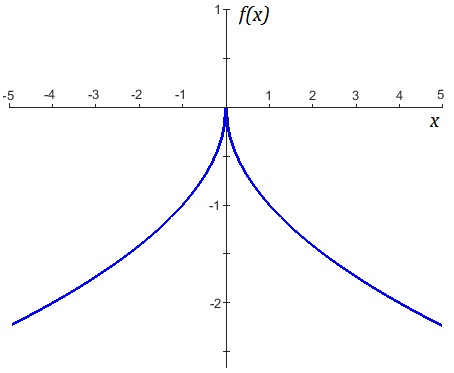### Example Question #3 : How To Find Continuous On The Interval By Graphing Functions

On what interval is the derivative of the function: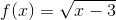continuous?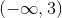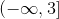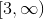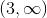The function is not continuous.Explanation:

The derivative of the funtion using the power rule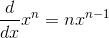is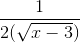,

so it is not continuous when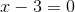or is negative.

This occurs when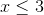, so the interval of continuity will be when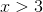, which is the interval.

### Example Question #4 : How To Find Continuous On The Interval By Graphing Functions

For what value(s) of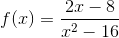?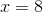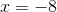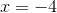Explanation:

For rational expressions, points of discontinuity often occur when the denomenator equals zero (triggering a division by zero).

Here we note that the denomenator will equal zero at positive and negative 4; however, we first must factor ensure that neither of these are factorable.

When factoring the rational expression the following occurs: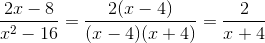We now see that the point x=4 was a removable discontinuity and in fact the only time this function is discontinous is at x=-4.

Recall: For a function to be continous at a point, the point must exist, the limit must exist, and the limit must equal the point.

### Example Question #5 : How To Find Continuous On The Interval By Graphing Functions

Evaluate the following limit: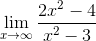Does not exist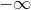Explanation:

The limit as any asymptotic function (a function with an asymptote) approaches infinity is always its horizontal asymptote.

To find the horizontal asymptote of a rational expression one must first look for the following conditions:

1. If the highest power of x is in the numerator then the horizontal asymptote does not exist.
2. If the highest power of x is in the denomenator then the horizontal asymptote is at 0.
3. If the highest power is in both, then divide the leading coefficents.

In the case of this problem the highest power is in both so we divide the leading coefficents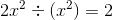Therefore, our limit is 2.